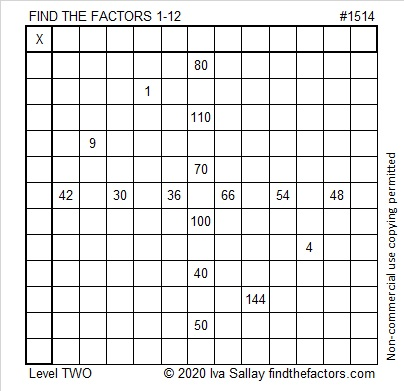# 1514 and Level 2

Contents

### Today’s Puzzle:

There is only one way to write the factors from 1 to 12 in both the first column and the top row so that this puzzle will behave like a multiplication table. The given clues will be the products of the factors you write. Can you find the way?### Factors of 1514:

• 1514 is a composite number.
• Prime factorization: 1514 = 2 × 757
• 1514 has no exponents greater than 1 in its prime factorization, so √1514 cannot be simplified.
• The exponents in the prime factorization are 1, and 1. Adding one to each exponent and multiplying we get (1 + 1)(1 + 1) = 2 × 2 = 4. Therefore 1514 has exactly 4 factors.
• The factors of 1514 are outlined with their factor pair partners in the graphic below.### More Facts about the number 1514:

1514 is the sum of two squares:
35² + 17² = 1514

1514 is the hypotenuse of a Pythagorean triple:
936-1190-1514 calculated from 35² – 17², 2(35)(17), 35² + 17²

This site uses Akismet to reduce spam. Learn how your comment data is processed.